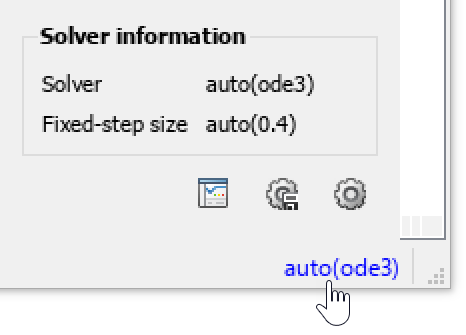# Fixed-step size (fundamental sample time)

Step size for fixed-step solver

Model Configuration Pane: Solver

## Description

When you use a fixed-step solver, you can specify the size of the fixed step the solver takes during simulation.

## Dependencies

To enable this parameter, set Periodic sample time constraint to `Unconstrained`.

## Settings

`auto` | positive scalar double
`auto`

By default, the fixed step size is `auto`. The software determines the appropriate step size for the simulation according to these rules:

• If the model includes periodic discrete sample times, the software chooses a step size equal to the greatest common divisor of the periodic sample times in the model. This step size ensures that the simulation takes a step for every sample time in the model.

• If the model does not include periodic discrete sample times and specifies a finite sample time, the solver chooses a step size that divides the simulation into 50 equal steps.

`${h}_{\mathrm{max}}=\frac{{t}_{stop}-{t}_{start}}{50}$`

• If the model does not include periodic sample times and specifies the stop time as `Inf`, the simulation uses a step size of `0.2`.

• If the model has no periodic sample times and uses Sine Wave or Signal Generator blocks, the software also considers the maximum frequency for the periodic signals generated by the source blocks. The step size is calculated to ensure that the step size is no smaller than one third of the minimum period of a periodic signal in the model, determined as the inverse of the maximum frequency $Fre{q}_{\mathrm{max}}$.

For a simulation with a finite stop time, if one third of the minimum period is smaller than the step size calculated to divide the simulation into fifty even steps, the simulation uses the step size determined using the maximum frequency.

`${h}_{\mathrm{max}}=\mathrm{min}\left(\frac{{t}_{stop}-{t}_{start}}{50},\left(\frac{1}{3}\right)\left(\frac{1}{Fre{q}_{\mathrm{max}}}\right)\right)$`

For a simulation with infinite stop time, if one third of the minimum period is smaller than `0.2`, the simulation uses the step size determined using the maximum frequency.

`${h}_{\mathrm{max}}=\mathrm{min}\left(\frac{{t}_{stop}-{t}_{start}}{50},\left(\frac{1}{3}\right)\left(\frac{1}{Fre{q}_{\mathrm{max}}}\right)\right)$`

• When the model is configured to start the simulation from an initial state specified as a `Simulink.op.ModelOperatingPoint` object, the software uses the fixed step size stored in the `ModelOperatingPoint` object.

When you allow the solver to determine the fixed step size, you can see the value that the solver determines several ways:

• Once the model is compiled, the Solver information window and tooltip provide information about the solver and fixed step size. To see the solver information, click or pause on the solver information string in the lower-right corner of the Simulink® Editor.Several actions result in compiling the model, including updating the block diagram and simulating the model.

• When you use the `get_param` function to get the value of the `FixedStep` parameter, the function returns `'auto'` if the parameter value is specified as `auto`. Once the model is compiled, you can programmatically access the fixed step size chosen by the software using the `CompiledStepSize` argument for the `get_param` function.

`fixedStepSize = get_param("mdlName","CompiledStepSize");`

• When you return simulation results as a single simulation output object, the metadata in the `Simulink.SimulationOutput` object includes the fixed step size used in the simulation. The fixed step size is stored as part of the model information in the `Simulink.SimulationMetadata` object, in the solver information.

```simMetadata = getSimulationMetadata(out); simModelInfo = simMetadata.ModelInfo; simSolverInfo = simModelInfo.SolverInfo; simFixedStep = simSolverInfo.FixedStepSize;```

positive scalar double

To use a value other than `auto`, specify the fixed step size in seconds as a double-precision value.

The specified step size must be less than or equal to the smallest sample time in the model and must be a factor of every sample time in the model.

When you configure a referenced model to use a local solver, the step size for the local solver must be an integer multiple of the step size for the parent solver. For more information, see Use Local Solvers in Referenced Models.

## Recommended Settings

The table summarizes recommended values for this parameter based on considerations related to code generation.

ApplicationSetting
DebuggingNo impact
TraceabilityNo impact
EfficiencyNo impact
Safety precautionNo impact

## Programmatic Use

 Parameter: `FixedStep` Type: string | character vector Value: `'auto'` | positive scalar number Default: `'auto'` Parameter: `CompiledStepSize` Type: string | character vector Value: positive scalar number

## Version History

Introduced before R2006a